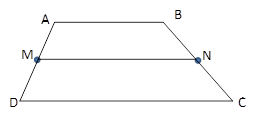Chapter 4.4, Problem 16E### Elementary Geometry for College St...

6th Edition
Daniel C. Alexander + 1 other
ISBN: 9781285195698

#### Solutions

Chapter
Section### Elementary Geometry for College St...

6th Edition
Daniel C. Alexander + 1 other
ISBN: 9781285195698
Textbook Problem
1 views

# In Exercises 11 to 16, the drawing shows trapezoid ABCD with A B ¯ | | D C ¯ ; also, M and N are midpoints of A D ¯ and B C ¯ , respectively.Exercises 11-16Given: A B = x + 3 y + 4 and D C = 3 x + 5 y − 2 .Find: MN, in terms of x         a n d         y .

To determine

To Find:

MN in terms of xandy for the trapezoid ABCD.

Explanation

Consider the following trapezoid ABCD.

Given:

AB=x+3y+4 and DC=3x+5y2.

Also in the given trapezoid, AB¯||DC¯.

M is the midpoint of the side AD¯ and N is the midpoint of the side BC¯.

MN is the median of the trapezoid ABCD

### Still sussing out bartleby?

Check out a sample textbook solution.

See a sample solution

#### The Solution to Your Study Problems

Bartleby provides explanations to thousands of textbook problems written by our experts, many with advanced degrees!

Get Started